Drive de adds rolex uk new Cartier series, new moon phase rolex replica watches equipped with Cartire 1904-LU MC refining workshop movement, 6 position with fake omega moon phase display and the complex function of accurate reproduction of the fake hublot watches new moon, moon, moon and moon cycle replacement time.
Summer Olympics @ Lift Up: Search Results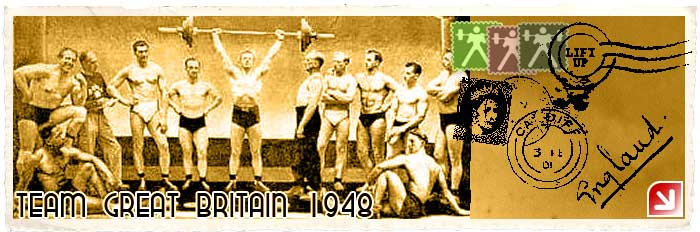## Lift Up: All-Time BestThe Lift Up project is brought to you by chidlovski.com.

## Olympic Legends @ Lift Up

K. Kangasniemi, Finland

# Summer Olympics since 19201972 Summer Olympics
Location: Munich, FRG
Weight Class: Middleweight  [75 kg]

Rank   Athlete Results (kg)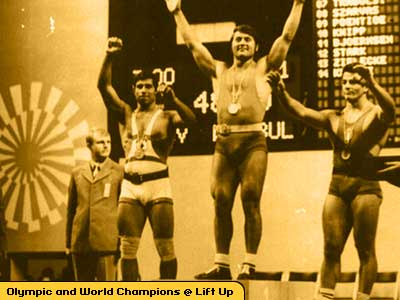1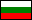Yordan BIKOV, Bulgaria 160 + 140+ 185 = 485WR
2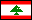Mohamed TRABOULSI, Lebanon 160 + 140+ 172.5 = 472.5
3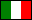Anselmo SILVINO, Italy 155 + 140+ 175 = 470
4Ondrej HEKEL , Czechoslovakia 150 + 142.5+ 170 = 462.5
5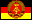Frank ZIELECKE, East Germany 150 + 140+ 170 = 460
6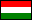Gabor SZARVAS, Hungary 150 + 135+ 175 = 460
7Andras STARK, Hungary 152.5 + 137.5+ 170 = 460
8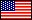Russel KNIPP, United States 160 + 127.5+ 170 = 457.5
9Frederick LOWE, United States 147.5 + 135+ 175 = 457.5
10Tore BJORNSEN, Norway 145 + 130+ 165 = 440
11Salvatore LAUDANI, Italy 155 + 120+ 160 = 440
12Christos SPYROU, Greece 145 + 117.5+ 167.5 = 430
13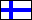Arvo ALA-PONTIO, Finland 145 + 122.5+ 160 = 427.5
14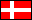Erling JOHANSEN, Denmark 137.5 + 127.5+ 160 = 425
15Aime TERME, France 127.5 + 142.5+ 155 = 425
16Ismail BAYRAM, Turkey 137.5 + 122.5+ 152.5 = 412.5
17Tony EBERT , New Zealand 137.5 + 117.5+ 155 = 410
18Natsagiin SER-OD, Mongolia 130 + 122.5+ 147.5 = 400
19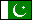Mohammad Malik ARSHAD, Pakistan 117.5 + 107.5+ 147.5 = 372.5
900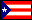Alfonso RODRIGUEZ, Puerto Rico 0+ 0 = 0
900Leopold PICHLER, Austria 145 + 0+ 0 = 0
900Keith ADAMS, Canada 137.5 + 0+ 0 = 0
900Werner DITTRICH, East Germany 0+ 0 = 0
900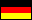Uwe KLICHE, West Germany 145 + 0+ 0 = 0
900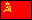Vladimir KANYGIN, Soviet Union 165 + 0+ 0 = 0
900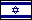Jossef ROMANO, Israel 0+ 0 = 0

Back to Search Tournaments

Top